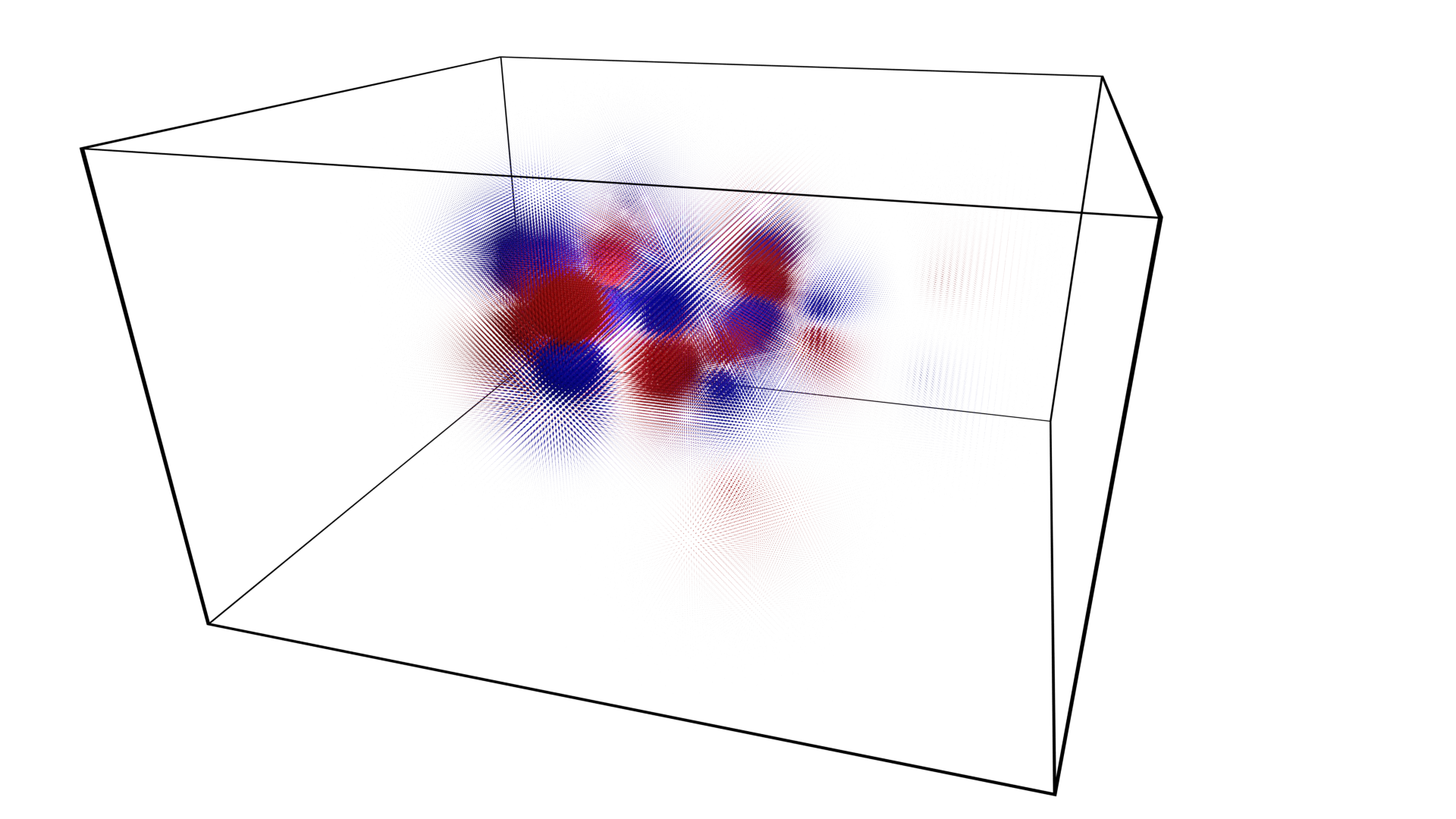# Cubefile

Cube files are generated from quantum mechanical chemistry calculations. They contain data about the atoms of a molecule: their element, charge and position, as well as volume data from molecular orbital calculations - where you can expect to find electrons within a molecule.

This package contains a single module for processing cube files. Example usage:

```>>> import Cubefile
>>> cf = Cubefile.Cubefile("_testfiles/caffeine_54.cube")
>>> cf.voxels.shape
(111, 98, 64)
```

The _testfiles directory includes selected cube files of molecular orbitals calculated for caffeine at B3LYP/6-31G(d) using the psi4 computational chemistry program.

The above file can be rendered as a point cloud, with red representing positive values; blue representing negative values and points scaled by a factor of 3.0.Cubefile file format reference: http://paulbourke.net/dataformats/cube/.

BOHR_TO_ANGSTROM: float = 0.529177210903

Conversion from Bohr to Angstrom. 2018 CODATA.

class Cubefile(data_source: Optional[Any] = None)[source]

Cubefile information.

Parameters:

data_source – Passed to `read()` if truthy.

property atom_count: int

Number of atoms.

atoms: List[Dict[str, Union[int, float, ndarray[Any, dtype[float64]]]]]

List of atom information.

Atom information is stored as `dict` with keys:
• “element”: atomic number [int]

• “charge”: charge [float]

• “xyz”: atomic coordinates (Å) [NDArray[float_type]]

filename: Optional[str]

The file name of the data file or `None`.

property max_voxel_val: float

Maximum absolute voxel value.

origin: ndarray[Any, dtype[float64]]

Origin of coordinate system used (Å).

Read a cubefile using `read_iterator()`.

Param:
1. If `os.path.isfile(data_source)` then the file is opened with `open()` and loaded. This sets the `filename` attribute.

2. If `data_source` is a `str` then the `data_source` is loaded using `splitlines()`.

3. If `data_source` is an `Iterator` then its contents are loaded.

Raises:

ValueError – if the `data_source` could not be loaded.

Read cube data from an iterator. See also: `read()`.

File format reference: http://paulbourke.net/dataformats/cube/.

Parameters:

iterator (Iterator[str]) – An iterator that yields cubefile data line by line as str.

Raises:
• ValueError – if the amount of voxel data is incorrect.

• ValueError – for parsing errors.

• ValueError – if non-square voxels are encountered.

reset() None[source]

Initialize object variables.

scale: ndarray[Any, dtype[float64]]

Scale.

unit_conversion: ndarray[Any, dtype[float64]]

Scaling of units that has been applied relative to Å.

property voxel_count: Tuple[int, ...]

Number of voxels in each dimension. Alias of `voxels.shape`

voxel_shape: ndarray[Any, dtype[float64]]

Shape of each voxel per dimension.

property voxel_total: int

Total number of voxels. Alias of `voxels.size`

voxels: ndarray[Any, dtype[float64]]

Voxel data. A 3-dimensional numpy array.

float_type

The `dtype` for all new numpy arrays. [numpy.float64]